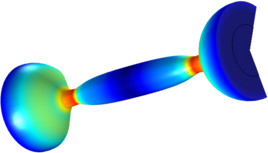# Bibliothèque d'Applications

#### Jet Instability — Moving Mesh

Application ID: 4650

The Marangoni effect results in a slip velocity in the tangential direction on a fluid/fluid interface due to gradients in the surface tension coefficient. When the surface tension coefficient is constant, a two-fluid system may exist in static equilibrium. This is because the surface tension force may be exactly balanced by a jump in the pressure across the interface. The pressure is discontinuous across the interface, but the velocity field is zero everywhere. The presence of a gradient in the surface tension coefficient means that the flow must be non-stationary. This is due to the fact that any force arising from the variability of the surface tension coefficient acts only in the tangential direction on the interface. This must be balanced by viscous forces which are only present in a moving fluid. In this example, an initially stationary, infinitely long liquid jet breaks up due to a spatially varying surface tension coefficient.This model example illustrates applications of this type that would nominally be built using the following products: